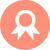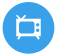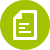## Servlet## WS## Hibernate# java8新特性之StreamApi Stream流中止操作

## stream流常用方法简介

```/**
* 查找与匹配
*
* allMatch:检查是否匹配所有元素
* anyMatch:检查是否至少匹配一个元素
* noneMatch:检查是否没有匹配所有元素
* findFirst:返回第一个元素+
* findAny:返回当前流中的任意元素
* count:返回流中元素的总和
* max:返回流中的最大值
* min:返回流中的最小值
*/
```
```@Test
public void test10() {
boolean b = list.stream()
/**检查是否匹配所有元素   就是检查对象中的元素是否都满足allMatch()里面的要求,是就返回true   反之 false*/
.allMatch( (x) -> x.getId()>2 );
System.out.println(b);

boolean b1 = list.stream()
/**检查是否至少匹配一个元素   就是检查对象中的元素是否至少有一个满足anyMatch()里面的要求,是就返回true   反之 false*/
.allMatch( (x) -> x.getId()>0 );
System.out.println(b1);

boolean b2 = list.stream()
/**检查是否没有匹配所有元素   就是检查对象中的元素是否有满足noneMatch()里面的要求,是就返回true   反之 false*/
.allMatch( (x) -> x.getId()>0 );
System.out.println(b2);

Optional b3 = list.stream()
/**先排序*/
.sorted((x,y) -> Double.compare( y.getId(),x.getId() ))
/**返回第一个元素*/
.findFirst( );
System.out.println(b3.get());

Optional optional = list.stream()
.filter( (x) -> x.getId()>4 )
/**findAny - 返回当前流中的任意元素*/
.findAny();
System.out.println(optional.get());

Long l = list.stream()
.filter( (x) -> x.getId()>0 )
/*** * count - 返回流中元素的总和*/
.count();
System.out.println(l);

Optional optional2 = list.stream()
/**max - 返回流中的最大值**/
.max( (x,y) -> Double.compare( x.getId(),y.getId() ) );
System.out.println(optional2.get());

Optional optional3 = list.stream()
/**min - 返回流中的最小值**/
.max( (x,y) -> Double.compare( x.getId(),y.getId() ) );
System.out.println(optional3.get());

/**
* 得到具体的最小id
*/
Optional<Integer> o = list.stream()
//首先通过映射,得到一个id流,这个流包含所有的id
.map( (x) -> x.getId() )
//从新建的流中得到
.min( (x,y) -> Double.compare( x,y ) );
System.out.println(o.get());
}
```

#### 好文推荐

Java265.com

http://www.java265.com

### 站长统计|粤ICP备14097017号-3